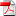# L2 Density Estimation Under Constraints

1
Sep

### Christian Musso, Nadia Oudjane

This paper presents a general methodology to estimate a probability density under linear constraints (on the support, bounded moments or quantiles,. . . ). The proposed approximation is the projection of the free density estimation on the set of the probability densities satisfying the constraints. In some cases, the solution of this projection problem can be expressed in a simple parametric form as a function of the free density estimate.

Plus d'infos.

•rr-fime-09-03